Published: 20 April 2018

# Consumption prediction of bearing spare parts based on a hybrid model

Yukun Chen1
Qi Gao2
Xiaobo Su3
Chenchen Yu4
1, 2, 3Shijiazhuang Division of Army Engineering University, Shijiazhuang, China
3Shijiazhuang Division of Army Infantry College, Shijiazhuang, China
4The Third Office of Troop 63916, Beijing, China
Corresponding Author:
Yukun Chen
Views 43

#### Abstract

Aiming at improving the accuracy of consumption prediction, a hybrid model was constructed, which designs an empirical wavelet filter bank to remove noise factors in original data. Besides the value prediction, the EWT-PGPR model can also give a certain credible interval, which effectively improves the practicability of the model.

## 1. Introduction

The noise factor can reduce the prediction accuracy deeply. The recovery of equipment operational capability is closely related to equipment maintenance support, and in which, bearing spare parts play an important role in equipment maintenance support. The accurate prediction of the consumption of bearing spare parts can meet the requirements of equipment maintenance support under the limited funds. The neural network grey theory  and support vector machine  and lots of other consumption forecasting methods were established. However, the consumption of bearing spare parts generally presents non-stationary characteristics due to multiple factors. Without considering the noise caused by the special circumstances such as operator error, the prediction methods of the bearing spare parts consumption often analyzed directly by the original data. In order to improve the situation, the empirical wavelet transform (EWT) was established to denoise the consumption series, which makes up for the lack of adaptive data processing ability of wavelet transform [3, 4] and the need of selecting wavelet basis in advance. Furthermore, the prediction accuracy of non-stationary time series can be effectively improved by discarding the influence of noise in original data.

Besides, the Partial autocorrelation-Gauss regression model (PGPR) was utilized to predict the consumption of bearing spare parts, which possesses good adaptability and strong versatility. Compared with the prediction methods such as neural network and support vector machine, the PGPR model has better adaptive parameter estimation and flexible nonparametric inference ability.

## 2. Empirical wavelet transform

Although the wavelet transform has a good denoising capability, but due to the need of selecting the wavelet basis in advance and the lack of adaptive data processing capabilities, the practical application of wavelet transform has been restricted. Therefore, the empirical wavelet transform method was established. Since the wavelet basis is constructed directly based on the information in the original time series, the empirical wavelet transform has better self-adaptability.

The empirical scaling function is defined as follow:

1
${\stackrel{^}{\varphi }}_{n}\left(\omega \right)=\left\{\begin{array}{l}1,\mathrm{}\mathrm{}\mathrm{}\mathrm{}\mathrm{}\mathrm{}\left|\omega \right|\le \left(1-\gamma \right){\omega }_{n},\\ \mathrm{c}\mathrm{o}\mathrm{s}\left[\frac{\pi \beta }{2}\left(\frac{\left|\omega \right|}{2{\tau }_{n}}-{\omega }_{n}+{\tau }_{n}\right)\right],\left(1-\gamma \right){\omega }_{n}\le \left|\omega \right|\le \left(1+\gamma \right){\omega }_{n},\\ 0,\mathrm{}\mathrm{}\mathrm{}\mathrm{}\mathrm{}\text{otherwise}\text{.}\end{array}\right\$

And the empirical wavelet is defined as:

2
${\stackrel{^}{\phi }}_{n}\left(\omega \right)=\left\{\begin{array}{l}1,\mathrm{}\mathrm{}\mathrm{}\mathrm{}\mathrm{}\left(1+\gamma \right){\omega }_{n}\le \left|\omega \right|\le \left(1+\gamma \right){\omega }_{n+1},\\ \mathrm{c}\mathrm{o}\mathrm{s}\left[\frac{\pi \beta }{2}\left(\frac{\left|\omega \right|}{2{\tau }_{n+1}}-{\omega }_{n+1}+{\tau }_{n+1}\right)\right],\left(1-\gamma \right){\omega }_{n+1}\le \left|\omega \right|\le \left(1-\gamma \right){\omega }_{n+1},\\ \mathrm{s}\mathrm{i}\mathrm{n}\left[\frac{\pi \beta }{2}\left(\frac{\left|\omega \right|}{2{\tau }_{n}}-{\omega }_{n}+{\tau }_{n}\right)\right],\left(1-\gamma \right){\omega }_{n}\le \left|\omega \right|\le \left(1-\gamma \right){\omega }_{n},\\ 0,\mathrm{}\mathrm{}\mathrm{}\mathrm{}\mathrm{}\text{otherwise}\text{,}\end{array}\right\$

where $\beta \left(x\right)$ is an arbitrary function satisfies the following conditions:

3
$\begin{array}{l}\beta \left(x\right)=\left\{\begin{array}{l}0,\mathrm{}\mathrm{}\mathrm{}\mathrm{}\mathrm{}x\le 0,\\ 1,\mathrm{}\mathrm{}\mathrm{}\mathrm{}\mathrm{}x\ge 1,\end{array}\right\\\ \beta \left(x\right)+\beta \left(1-x\right)=1,\mathrm{}\mathrm{}\mathrm{}\mathrm{}\mathrm{}0

The correlation coefficients can be calculated after the empirical wavelet basis function is determined, in which the approximation coefficient ${W}_{f}^{\epsilon }\left(0,t\right)$ is obtained from the inner product between empirical scaling function and signal:

4
${W}_{f}^{\epsilon }\left(0,t\right)=⟨f,{\varphi }_{1}⟩=\int f\left(\tau \right)\overline{{\varphi }_{1}\left(\tau -t\right)}d\tau =f\left(\tau \right){\left[\overline{{\stackrel{^}{\varphi }}_{1}\left(\tau -t\right)}\right]}^{\vee }.$

The detail coefficient ${W}_{f}^{\epsilon }\left(n,t\right)$ is empirical wavelets and the inner product of signals:

5
${W}_{f}^{\epsilon }\left(n,t\right)=⟨f,{\phi }_{n}⟩=\int f\left(\tau \right)\overline{{\phi }_{n}\left(\tau -t\right)}d\tau =f\left(\tau \right){\left[\overline{{\stackrel{^}{\phi }}_{n}\left(\tau -t\right)}\right]}^{\vee },$

where $\vee$ represents Fourier inverse transformation.

Then the reconstructed signal is:

6
$f\left(t\right)={W}_{f}^{\epsilon }\left(0,t\right){\varphi }_{1}\left(t\right)+\sum _{n=1}^{N}{W}_{f}^{\epsilon }\left(n,t\right){\phi }_{n}\left(t\right)$
$\mathrm{}\mathrm{}\mathrm{}\mathrm{}\mathrm{}\mathrm{}={\left[{\stackrel{^}{W}}_{f}^{\epsilon }\left(0,\omega \right){\varphi }_{1}\left(\omega \right)+\sum _{n=1}^{N}{\stackrel{^}{W}}_{f}^{\epsilon }\left(n,\omega \right){\phi }_{n}\left(\omega \right)\right]}^{\vee },$

and the corresponding modal functions are as follows:

7
${f}_{0}\left(t\right)={W}_{f}^{\epsilon }\left(0,t\right){\varphi }_{1}\left(t\right),$
8
${f}_{k}\left(t\right)={W}_{f}^{\epsilon }\left(k,t\right){\phi }_{k}\left(t\right).$

## 3. Prediction method of consumption

The general structure of the prediction method which includes two stages was shown in Fig. 1.

Stage 1: Firstly, the original data is extended, and when the condition satisfied, the filter bank is established to filter series of the consumption for extracting the subseries. Thus, the modes and one residual are obtained. The residual here is the noise in the original consumption series.

Stage 2: Firstly, the PACF is utilized to identify the coefficient. And then construct the Gaussian likelihood function. The gradient optimization method is applied to the function, and we can obtain the maximum parameter of the posterior estimation. Finally, the predicted value as well as the interval of consumption can be obtained by GPR.

Fig. 1The overall framework of consumption forecasting of equipment maintain bearing spare parts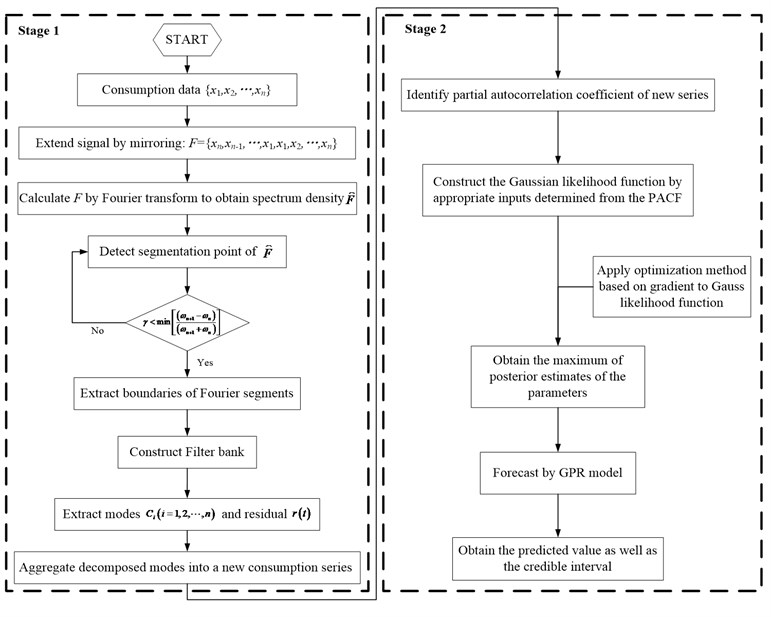## 4. Numerical example

The consumption of spare part from  was used as original data to analyze.

Table 1The consumption data of spare part in 2006 to 2015

 Time 2006 2007 2008 2009 2010 2011 2012 2013 2014 2015 Consumption 38 44 47 41 36 32 25 27 39 40

### 4.1. Data analysis

As the consumption series of spare part is non-stationary. The data from 2006 to 2013 were used as input.

### 4.2.1. Data preprocessing

The original consumption series was decomposed into 3 uncorrelated modes by the EWT algorithm, which including a residual as shown in Fig. 2. Then, the two modes after denoising were reconstructed by EWT, and the de-noised data was obtained.

Fig. 2The series of consumption decomposed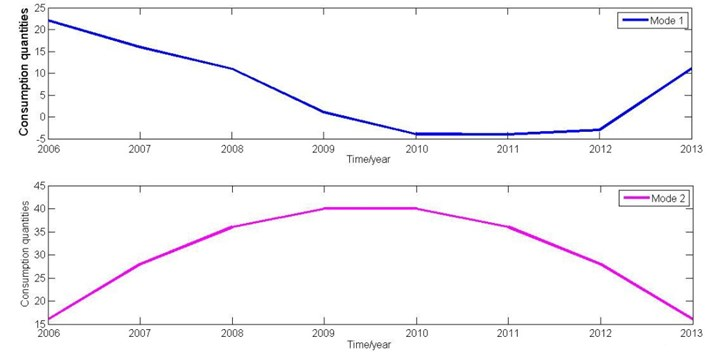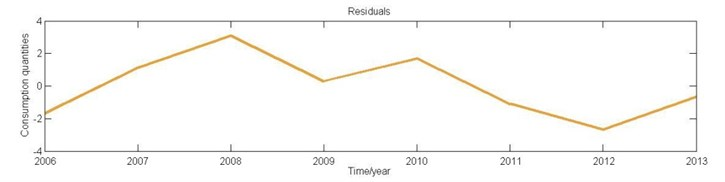Fig. 3The comparison between original and de-noised data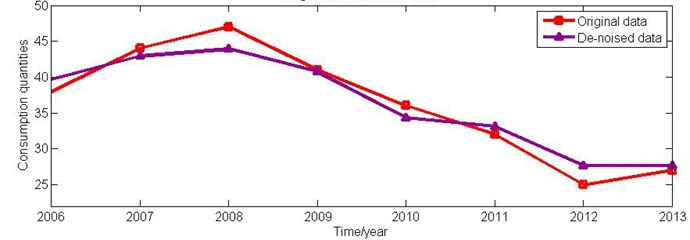### 4.2.2. Forecasting

Firstly, the partial autocorrelation function of the consumption series after denoising was calculated, and the results were shown in Fig. 4. It can be seen from the graph that the partial autocorrelation coefficient of the consumption series after reconstruction is high.

Fig. 4The PACF values of consumption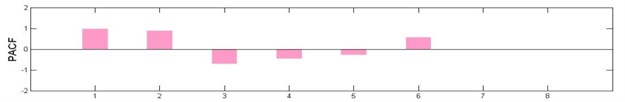After removing the internal correlation of the series, the first 3 lag of PACF are significant, which has a certain impact to consumption prediction. Then, Bayesian inference was performed based on the super parameter of the GPR model which has been defined previously. Finally, the Gauss model was used to predict the future consumption of the series. The consumption forecast values and forecast intervals of 2014 and 2015 were shown in Fig. 5.

Fig. 5The actual data and predicting series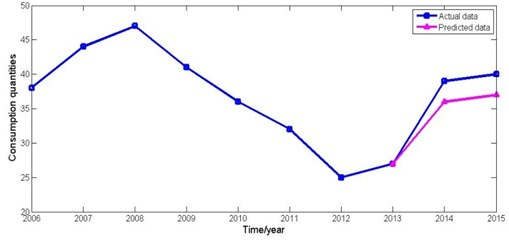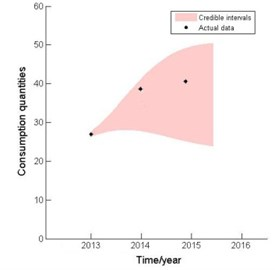### 4.3. Comparisons and discussion

It can be seen from Fig. 5 that the actual consumption of the bearing spare parts from 2014 to 2015 is in the prediction range derived from the established model, and the consumption trend of the prediction results is similar to the actual situation. In addition, due to the prediction value and interval can be given simultaneously, the hybrid model can provide more guidance for equipment managers, such as selecting the reserve of bearing spare parts according to the predicted value, maximum value and minimum value.

## 5. Conclusions

In this article, a hybrid model to forecast bearing spare parts consumption was proposed. The moving window is used to process the training data in order to adapt to the time-varying of consumption series. Besides the value prediction, the EWT-PGPR model can also give a certain credible interval, which effectively improves the practicability of the model.